The magnetic field due to a short magnet at a point on its axis at distance X cm from the middle point of the magnet is 200 Gauss. The magnetic field at a point on the neutral axis at a distance X cm from the middle of the magnet is

1. 100 Gauss               2. 400 Gauss
3. 50 Gauss                 4. 200 Gauss

Concept Questions :-

Analogy between electrostatics and magnetostatics
High Yielding Test Series + Question Bank - NEET 2020

Difficulty Level:

A sensitive magnetic instrument can be shielded very effectively from outside magnetic fields by placing it inside a box of
1. Teak wood
2. Plastic material
3. Soft iron of high permeability
4. A metal of high conductivity

Concept Questions :-

Magnetization and magnetic intensity
High Yielding Test Series + Question Bank - NEET 2020

Difficulty Level:

A uniform magnetic field, parallel to the plane of the paper existed in space initially directed from left to right. When a bar of soft iron is placed in the field parallel to it, the lines of force passing through it will be represented by

1.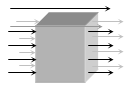2.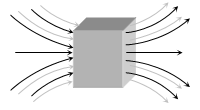3.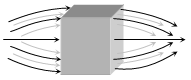4.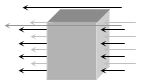Concept Questions :-

Magnetic materials
High Yielding Test Series + Question Bank - NEET 2020

Difficulty Level:

A neutral point is obtained at the centre of a vertical circular coil carrying current. The angle between the plane of the coil and the magnetic meridian is :

a) 0                           (b) 45°

(c) 60°                       (d) 90°

Concept Questions :-

Earth's magnetism
High Yielding Test Series + Question Bank - NEET 2020

Difficulty Level:

The figure below shows the north and south poles of a permanent magnet in which n turn coil of the area of cross-section A is resting, such that for a current i passed through the coil, the plane of the coil makes an angle $\theta$ with respect to the direction of magnetic field B. If the plane of the magnetic field and the coil are horizontal and vertical respectively, the torque on the coil will be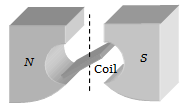(a)τ = $niAB\mathrm{cos}\theta$

(b)τ = $niAB\mathrm{sin}\theta$

(c)τ=niAB

(d) None of the above, since the magnetic field is radial

Concept Questions :-

Bar magnet
High Yielding Test Series + Question Bank - NEET 2020

Difficulty Level:

Points A and B are situated perpendicular to the axis of a 2cm long bar magnet at large distances X and 3X from its centre on opposite sides. The ratio of the magnetic fields at A and B will be approximately equal to
(a) 1 : 9               (b) 2 : 9
(c) 27 : 1             (d) 9 : 1

Concept Questions :-

Bar magnet
High Yielding Test Series + Question Bank - NEET 2020

Difficulty Level:

Two short magnets with their axes horizontal to the magnetic meridian are placed with their centres 40 cm east and 50 cm west of the magnetic needle. If the needle remains undeflected, the ratio of their magnetic moments  is

(a) 4:5

(b) 16:25

(c) 64:125

(D) 2:$\sqrt{5}$

Concept Questions :-

Bar magnet
High Yielding Test Series + Question Bank - NEET 2020

Difficulty Level:

If a bar magnet of magnetic moment M is freely suspended in a uniform magnetic field of strength B, the work done in rotating the magnet through an angle is

1. $MB\left(1-\mathrm{sin}\theta \right)$

2. $MB\mathrm{sin}\theta$

3. $MB\mathrm{cos}\theta$

4. $MB\left(1-\mathrm{cos}\theta \right)$

Concept Questions :-

Bar magnet
High Yielding Test Series + Question Bank - NEET 2020

Difficulty Level:

Two small bar magnets are placed in a line with like poles facing each other at a certain distance d apart. If the length of each magnet is negligible as compared to d, the force between them will be inversely proportional to

(a) d

(b) d2

(c) $\frac{1}{{d}^{2}}$

(d) ${d}^{4}$

Concept Questions :-

Bar magnet
High Yielding Test Series + Question Bank - NEET 2020

Difficulty Level:

A magnet of magnetic moment M is situated with its axis along the direction of a magnetic field of strength B. The work done in rotating it by an angle of 180o will be

(a) -MB                         (b) +MB

(c) 0                             (d) +2MB

Concept Questions :-

Bar magnet